## k-Nearest Neighbors algorithms

In this blog post, I am going to introduce one of the most intuitive algorithms in the field of Supervised Learning, the k-Nearest Neighbors algorithm (kNN).

# The original k-Nearest Neighbors algorithm

The kNN algorithm is very intuitive. Indeed, with the assumption that items close together in the dataset are typically similar, kNN infers the output of a new sample by first constructing the distance score with every sample in the training dataset. From there, it creates a ‘neighbor zone’ by selecting samples that are ‘near’ the candidate one, and does the supervised tasks based on samples lie inside that zone. The task could be either classification or regression.

Let’s start with the basic kNN algorithm. Letbe our training dataset withsamples belong toclasses, whereis the class of one sample, anddenotes the corresponding feature vector that describes the characteristics of that sample. Furthermore, it is necessary to define the suitable distance metric, since it drives the algorithm to select neighbors and make predictions later on. The distance metric, is a mappingover a vector space, where the following conditions are satisfied:

••••In the following steps to describe the k-Nearest Neighbors algorithm, the Euclidean distance will be used as the distance metric.

For any new instance:

• Findwhereis the set ofsamples that are closest to• The way to define the nearest neighbors is based on distance metric(Note that we are using Euclidean distance).• The classifieris defined as:whereis the unit function. Note that for the regression problem, the functionwill just an average of all response valuesfrom neighbor samples.

# Weighted k-Nearest Neighbors

In the kNN algorithm, we weigh all neighbors equally. It may affect the inference steps, especially when the neighbor zone becomes bigger and bigger. To strengthen the effect of ‘close’ neighbors than others, the weighted scheme of k-Nearest Neighbors is applied.

Weighted k-Nearest Neighbors is based on the idea that, within, such observations that are closer to, should get a higher weight than the further neighbors. Now it is necessary to note some properties of any weighting schemeson any distance metric:

•••decreases monotonously forFor any new instance:

• We findwhereis the set ofsamples that are closest to• Theth neighbor is used for standardization of thesmallest distance:• We transform the standardized distancewith any kernel functioninto weights.
• The classifieris defined as:# The pros and cons of kNN, and further topics

The kNN and weighted kNN do not rely on any specific assumption on the distribution of the data, so it is quite easy to apply it to many problems as the baseline model. Furthermore, kNN (and its family) is very intuitive for understanding and implementing, which again makes it a worthy try-it-first approach for many supervised problems.

Despite those facts, kNN still has challenges in some aspects: It is computationally expensive – especially when the dataset size becomes huge. Another challenge is choosing the ‘correct’ distance metric that best follows the assumption for using this algorithm: items close together in the data set should be typically similar. Lastly, the curse of dimensionality heavily affects the distance metric. Beyer et al. proves that, under some preconditions, in high dimension space, all points converge to the same distance from the query point. In this case, the concept of ‘nearest neighbors’ is no longer meaningful.

## Binomial Theorem

Can you expand on? I guess you would find that is quite easy to do. You can easily find that.

How about the expansion of. It is no longer easy.

It is no longer easy, isn’t it. However, if we use Binomial Theorem, this expansion becomes an easy problem.

Binomial Theorem is a very intriguing topic in mathematics and it has a wide range of applications.

# Theorem

Letbe real numbers (or complex, or polynomial). For any positive integer, we have:where,Proof:

We will use prove by induction. The base caseis obvious. Now suppose that the theorem is true for the case, that is assume that:we will need to  show that, this is true forLet us consider the left-hand side of the equation above## We can now apply Pascal’s identity:The equation above can be simplified to:as we desired.

### Example 1:  Power rule in Calculus

In calculus, we always use the power rule thatWe can prove this rule using the Binomial Theorem.

Proof:

Recall that derivative for any continuous function f(x) is defined as:Letbe a positive integer and letThe derivative of f(x) is:### Example 2:  Binomial Distribution

Let X be the number of Head a sequence of n independent coin tossing. X is usually model by binomial distribution in the probability model. Letbe the probability that a head shows up in a toss, and let. The probability that there ishead in the sequence oftoss is:We know that sum of all the probability must equal to 1. In order to show this, we can use Binomial Theorem. We have:Please also check another article Gaussian Samples and N-gram language models ,Bayesian model, Monte Carlo for statistics knowledge.

# Hiring Data Scientist / Engineer

We are looking for Data Scientist and Engineer.

# Vietnam AI / Data Science LabPlease also visit Vietnam AI Lab

## Bayesian estimator of the Bernoulli parameter

In this post, I will explain how to calculate a Bayesian estimator. The taken example is very simple: estimate the parameter θ of a Bernoulli distribution.

A random variable X which has the Bernoulli distribution is defined as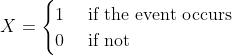with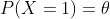In this case, we can write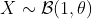.

In reality, the simplest way to estimate θ is to sample X, count how many times the event occurs, then estimate the probability of occurring events. This is exactly what the frequentists do.

In this post, I will show how do the Bayesian statisticians estimate θ. Although this doesn’t have a meaningful application, it helps to understand how do Bayesian statistics work. Let’s start.

# The posterior distribution of θ

Denote Y as the observation of the event. Given the parameter θ, if we sample the event n time, then the probability that the event occurs k time is (this is called the probability density function of Bernoulli )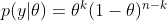In Bayesian statistics, we would like to calculate

By using the Bayesian formula, we haveWith the prior distribution of theta as a Uniform distribution, p(θ) = 1, and it is easy to prove thatwhere Γ is the Gamma distribution. Hence, the posterior distribution isFortunately, this is the density function of the Beta distribution:We use the following properties for evaluating the posterior mean and variance of theta.

If, then# Simulation

In summary, the Bayesian estimator of theta is the Beta distribution with the  mean and variance as above. Here are the Python codes for simulating data and estimating theta

def bayes_estimator_bernoulli(data, a_prior=1, b_prior=1, alpha=0.05):
'''Input:
data is a numpy array with binary value, which has the distribution B(1,theta)    a_prior, b_prior: parameters of prior distribution Beta(a_prior, b_prior)    alpha: significant level of the posterior confidence interval for parameter    Model:
for estimating the parameter theta of a Bernoulli distribution    the prior distribution for theta is Beta(1,1)=Uniform[0,1]    Output:
a,b: two parameters of the posterior distribution Beta(a,b)
pos_mean: posterior estimation for the mean of theta
pos_var: posterior estimation for the var of theta'''
n = len(data)
k = sum(data)
a = k+1
b = n-k+1
pos_mean = 1.*a/(a+b)
pos_var = 1.*(a*b)/((a+b+1)*(a+b)**2)
## Posterior Confidence Interval
theta_inf, theta_sup = beta.interval(1-alpha,a,b)
print('Prior distribution: Beta(%3d, %3d)' %(a_prior,b_prior))
print('Number of trials: %d, number of successes: %d' %(n,k))
print('Posterior distribution: Beta(%3d,%3d)' %(a,b))
print('Posterior mean: %5.4f' %pos_mean)
print('Posterior variance: %5.8f' %pos_var)
print('Posterior std: %5.8f' %(np.sqrt(pos_var)))
print('Posterior Confidence Interval (%2.2f): [%5.4f, %5.4f]' %(1-alpha, theta_inf, theta_sup))
return a, b, pos_mean, pos_var

# Example
n = 129 # sample size
data = np.random.binomial(size=n, n=1, p=0.6)
a, b, pos_mean, pos_var = bayes_estimator_bernoulli(data)

And the result is

Prior distribution: Beta(  1,   1)
Number of trials: 129, number of successes: 76
Posterior distribution: Beta( 77, 54)
Posterior mean: 0.5878
Posterior variance: 0.00183556
Posterior std: 0.04284341
Posterior Confidence Interval (0.95): [0.5027, 0.6703]

In the simulation, we simulated 129 data from the Bernoulli distribution with θ=0.6. And the Bayesian estimation of θ is the posterior mean which is 0.5878.
This is a very simple example of Bayesian estimation. In reality, it is usually tricky to determine a closed-form solution of the posterior distribution from the given prior distribution. In that case, the Monte Carlo technique is one of the solutions to approximate the posterior distribution.
Please also check Gaussian Samples and N-gram language models for more statistics knowledge.

# Hiring Data Scientist / Engineer

We are looking for Data Scientist and Engineer.

# Vietnam AI / Data Science LabPlease also visit Vietnam AI Lab

# Background

The goal of this project is to generate Gaussian samples in 2-D from uniform samples, the latter of which can be readily generated using built-in random number generators in most computer languages.

In part 1 of the project, the inverse transform sampling was used to convert each uniform sample into respective x and y coordinates of our Gaussian samples, which are themselves independent standard normal (having mean of 0 and standard deviation of 1):

However, this method uses the inverse cumulative distribution function (CDF) of the Gaussian distribution, which is not well-defined. Therefore, we approximated this function using the simple Taylor series. However, this only samples accurately near the Gaussian mean, and under-samples more extreme values at both ends of the distribution.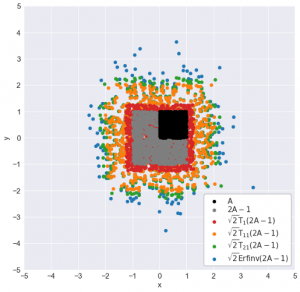A: left-side area (uniformly sampled). Tᵢ: the iᵗʰ-degree Taylor series approximation of the inverse Gaussian CDF: √2 Erfinv(2A-1)

In part 2 of the project, we used the Box-Muller transform, a more direct method to transform the uniform samples into Gaussian ones. The implementation of the algorithm is quite simple, as seen below, but its derivation requires some clever change of variables: instead of sampling Gaussian x and y coordinates for each point, we will sample a uniformly distributed angle (from 0 to 2π) and an exponentially-distributed random variable that represents half of a squared distance of the sample to the origin.

In this part of the project, I will present an even simpler method than the above two methods. Even better, this method is one that every statistics students are already familiar with.

# The Central Limit Theorem

It turns out, we can rely on the most fundamental principle of statistics to help us generate Gaussian samples: the central limit theorem. In very simple terms, the central limit theorem states that:

Given n independent random samples from the same distribution, their sum will converge to a Gaussian distribution as n gets large.

Therefore, to generate a Gaussian sample, we can just generate many independent uniform samples and add them together! We then repeat this routine to generate the next standard Gaussian sample until we get enough samples for our x-coordinates. Finally, we just repeat the same steps to generate the y coordinates.

Note that this method will work even if the samples that we start with are not uniform — they are Poisson-distributed, for example. This is because the central limit theorem holds for virtually all probability distributions *cough let’s not talk about the Cauchy distribution cough*.

# Generate Gaussian samples by central limit theorem

To generate, say, 1000 Gaussian sums (n_sums = 1000) where each is the sum of 100 uniform samples (n_additions = 100):

n_additions = 100
n_points = 1000# 0. Initialize random number generator
rng = np.random.RandomState(seed=24) # 1. Generate matrix of uniform samples
uniform_matrix = rng.uniform(size=(n_additions, n_points))# 2. Sum uniform elements down each column to get all Gaussian sums
gaussians = uniform_matrix.sum(axis=0)

We can apply the above method to generate Gaussian samples for each coordinate, using different random number generators for x and for y to ensure that the coordinates are independent of each other. Visualizing the intermediate sums after each addition, we see that:

# Background

In part 1 of this project, I’ve shown how to generate Gaussian samples using the common technique of inversion sampling:

The bad news: the Gaussian inverse CDF is not well-defined, so we have to approximate that function, and the simple Taylor series was used. However, this only samples accurately near the Gaussian mean, and under-samples more extreme values at both ends of the distribution.A: left-side area (uniformly sampled). Tᵢ: the iᵗʰ-degree Taylor series approximation of the inverse Gaussian CDF: √2 Erfinv(2A-1)

Therefore, in this part of the project, we will investigate a more “direct” sampling method that does not depend on the approximation of the Gaussian inverse CDF. This method is called the Box-Muller transform, after the two mathematicians who invented the method in 1958: the British George E. P. Box and the American Mervin E. Muller.

# How does the Box-Muller transform work?

For this project, my goal is to generate Gaussian samples in two dimensions i.e. generating samples whose x and y coordinates are independent standard normals (Gaussian with zero mean and standard deviation of 1). In part 1, I used the inverse Gaussian CDF to generate separately the x and y coordinates from their respective uniform samples (U₁ and U₂):

For the Box-Muller transform, I will also start with the same two uniform samples. However, I will transform these uniform samples into the x and y coordinates using much simpler formulas:

Despite the strong coupling between U₁ and U₂ in each of the two formulas above, the generated x and y coordinates, which are both standard Gaussians, are still surprisingly independent from each other! In the derivation of the Box-Muller transform that follows, I will demonstrate why this is indeed this case.

# Derivation of Box-Muller transform

We know that for any two independent random variables x and y, the joint probability density f(x, y) is simply the product of the individual density functions: f(x) and f(y). Furthermore, the Pythagoras theorem allows us to combine the x² and y² terms in each of the Gaussian density functions. This results in the -r²/2 term in the exponential of the joint distribution, where r is the distance from the origin to the 2-D Gaussian sample.

To make it simpler, we then define a variable s that is equal to r²/2. In other words, s is simply half of the squared distance from the origin of our Gaussian sample. Written this way, the joint PDF is simply the product of a constant (1/2π) and an exponential (e⁻ˢ).

# Background

Gaussian sampling — that is, generating samples from a Gaussian distribution — plays an important role in many cutting-edge fields of data science, such as Gaussian processvariational autoencoder, or generative adversarial network. As a result, you often see functions like tf.random.normal in their tutorials.

But, deep down, how does computer know how to generate Gaussian samples? This series of blog posts will show 3 different ways that we can program our computer (via Python) to do so. You will also see how R and Python generate Gaussian samples using modified versions of some of these methods.

# Starting point: the uniform number generator

Of course, we can’t generate Gaussian samples from thin air. Instead, we start with a random number generator that exists in almost all programming languages: the uniform random number generator. It generates a random number that could take any value between 0 and 1. For Python, the numpy.random module uses the Mersenne twister to generate a uniformly-distributed float that is in the interval [0.0, 1.0).

Since Gaussians are better visualized in 2 dimensions — we are all familiar with the Gaussian “blob” in the xy-plane — I will demonstrate the 3 sampling methods in 2-D, especially since one of the methods do generate Gaussians in two dimensions at the same time.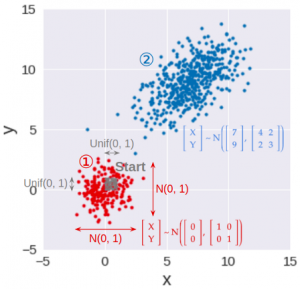Step 1: Generate standard Gaussian samples in 2-D. Step 2: Transform standard Gaussian samples to have given means, variances, and covariance between x and y

As a result, this series is broken down into 3 parts (see accompanying image):

# Method 1: Inverse transform sampling

This is the most basic, yet most common, way to convert a uniform random sample into a random sample of any distribution, including Gaussian. This method works by applying the inverse function of the Gaussian CDF (cumulative distribution function) to transform a uniform sample into a Gaussian sample.

To make sure that the Gaussian samples for the x- and y-coordinates are independent, we can use two different uniform samples, one for x (U₁), and one for y (U₂). These two uniform samples can be generated using two different random number generators (two different RandomState initialized by different seeds, for example) so that they are independent in the first place.

# How does this work?

This method works by exploiting a mind-blowing principle:

For any distribution, the cumulative probability is always uniformly distributed.

The arithmetic proof of this principle is straightforward but rather boring, and you can view it from this lecture. Instead, I will show the geometric interpretation of this principle. But first, let’s clarify what cumulative probability is:

# Background

In previous parts of my project, I built different n-gram models to predict the probability of each word in a given text. This probability is estimated using an n-gram — a sequence of words of length n — which contains the word. The below formula shows how the probability of the word “dream” is estimated as part of the trigram “have a dream”:

We train the n-gram models on the book “A Game of Thrones” by George R. R. Martin (called ). We then evaluate the models on two texts: “A Clash of Kings” by the same author (called ), and “Gone with the Wind” — a book from a completely different author, genre, and time (called ).

The metric to evaluate the language model is average log-likelihood: the average of the log probability that the model assigns to each word in the evaluation text.

Often, the log of base 2 is applied to each probability, as is the case in the first two parts of the project. Nevertheless, in this part, I will use a natural log, as it makes it simpler to derive the formulas that we will be using.

# Problem

In part 2, the various n-gram models — from unigram to 5-gram — were evaluated on the evaluation texts ( and , see graphs below).

From this, we notice that:

• The Bigram model performs slightly better than the unigram model. This is because the previous word to the bigram can provide important context to predict the probability of the next word.
• Surprisingly, the trigram model and up is much worse than bigram or unigram models. This is largely due to the high number of trigrams, 4-grams, and 5-grams that appear in the evaluation texts but nowhere in the training text. Hence, their predicted probability is zero.
• For most n-gram models, their performance is slightly improved when we interpolate their predicted probabilities with the uniform model. This seems rather counter-intuitive since the uniform model simply assigns equal probability to every word. However, as explained in part 1, interpolating with this “dumb” model reduces the overfit and variance of the n-gram models, helping them generalize better to the evaluation texts.

In this part of the project, we can extend model interpolation even further: instead of separately combining each n-gram model with the uniform, we can interpolate different n-gram models with one another, along with the uniform model.

# What to interpolate?

The first question to ask when interpolating multiple models together is:

To answer this question, we use the simple strategy outlined below:

1. First, we start with the uniform model. This model will have very low average log-likelihoods on the evaluation texts since it assigns every word in the text the same probability.
2. Next, we interpolate this uniform model with the unigram model and re-evaluate it on the evaluation texts. We naively assume that the models will have equal contribution to the interpolated model. As a result, each model will have the same interpolation weight of 1/2.
3. We then add the bigram model to the mix. Similarly, in this 3-model interpolation, each model will simply have the same interpolation weight of 1/3.
4. We keep adding higher n-gram models to the mix while keeping the mixture weights the same across model, until we reach the 5-gram model. After each addition, the combined model will be evaluated against the two evaluation texts,  and .

# Coding the interpolation

In part 2, each evaluation text had a corresponding probability matrix. This matrix has 6 columns — one for each model — and each row of the matrix represents the probability estimates of each word under the 6 models. However, since we want to optimize the model performance on both evaluation texts, we will vertically concatenate these probability matrices into one big evaluation probability matrix (803176 rows × 6 columns):